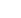# 4.3 Generating LL(1) Parsing Tables

Constructing LL(1) parsing tables is relatively easy (compared to constructing the tables for lexical analysis). The table is constructed using the following algorithm:

Algorithm

LL(1) Table Generation

``` For every production Ain the grammar:```

``` 1. Ifcan derive a string starting with a (i.e., for all a in FIRST() , ```

``` ```

``` Table [A, a] = A```
``` 2. Ifcan derive the empty string,, then, for all b that can follow a string derived from A (i.e., for all b in FOLLOW (A) , Table [A,b] = A```
``` ``` EXAMPLE 5 Constructing an LL(1) parse table entry using rule 1

Consider the production ETE' from the non-left-recursive, left-factored expression grammar in Example 4. FIRST(TE') = { (, Id }, so Table[E,(] = ETE' and Table[E,Id] = ETE'.
EXAMPLE 6 Constructing an LL(1) parse table entry using rule 2

Consider the production E'from the same grammar of Example 4. Since ";", ")" and "\$" are in FOLLOW (E'), this production occurs in the table in the row labeled E', and under the columns labeled ";", ")", and "\$".

LL(1) parsing tables may be generated automatically by writing procedures for FIRST () and FOLLOW(A). Then, when the grammar is input, A andare identified for each production and the two steps above followed to create the table.Send questions and comments to: Karen Lemone